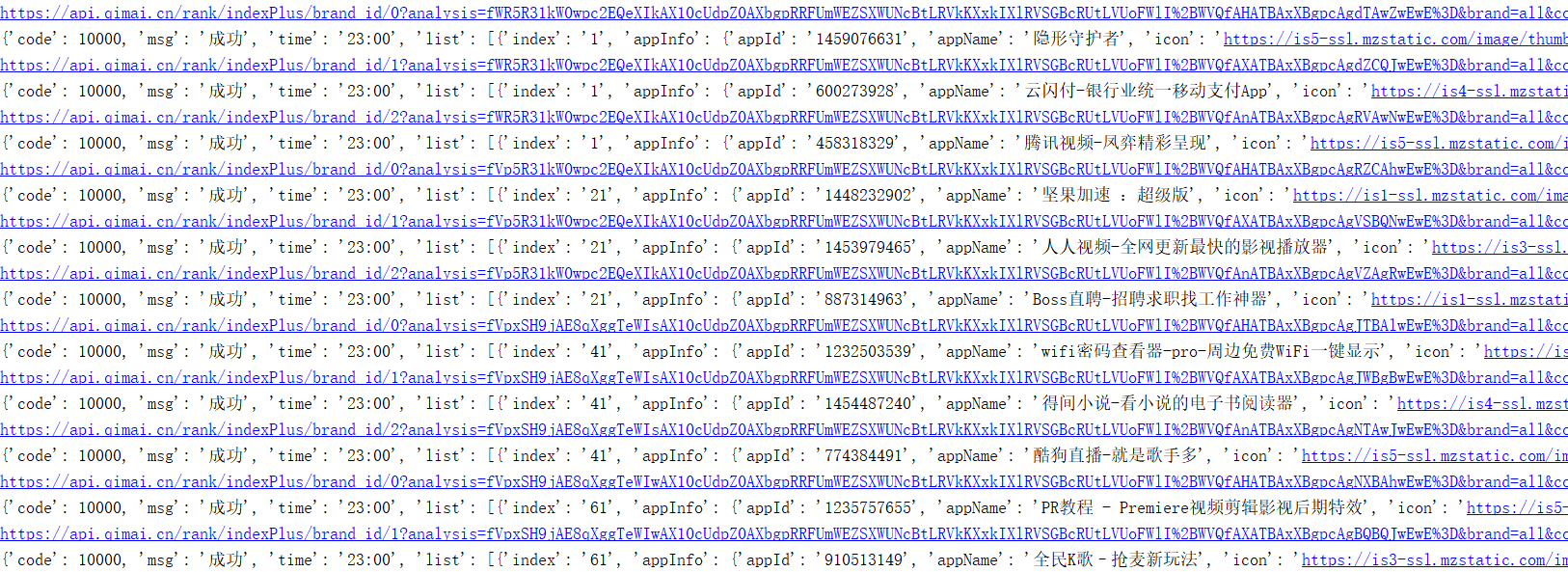# 数据爬取 js 分析：对加密参数进行 js分析

hresh 354## 二、页面分析

### 1.抓包分析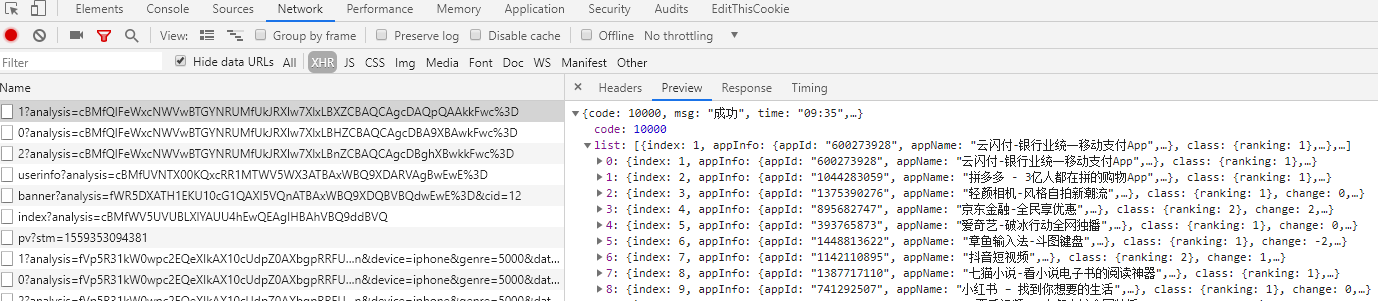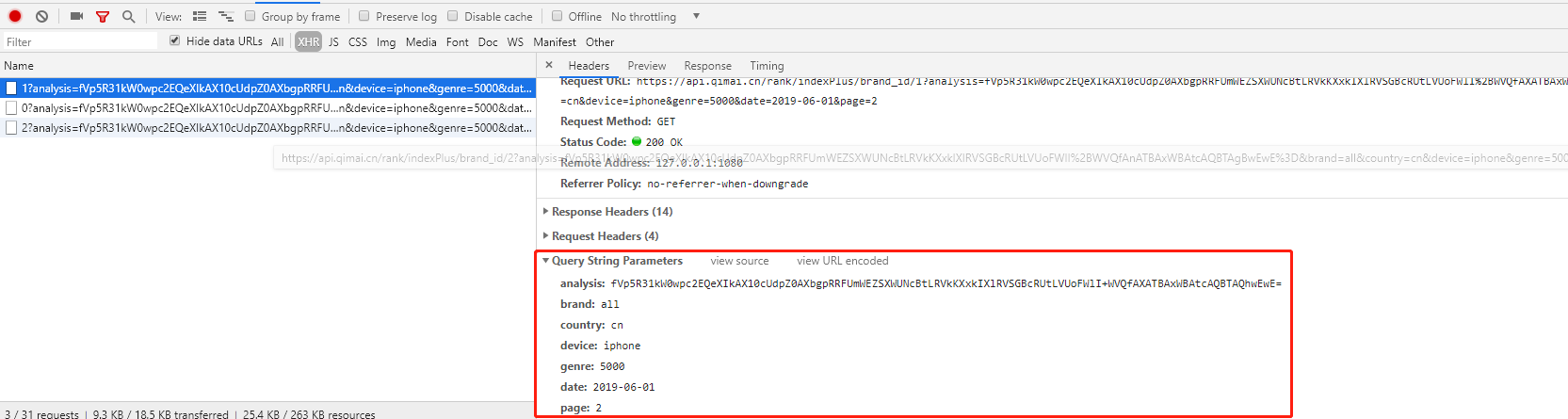### 2.调试分析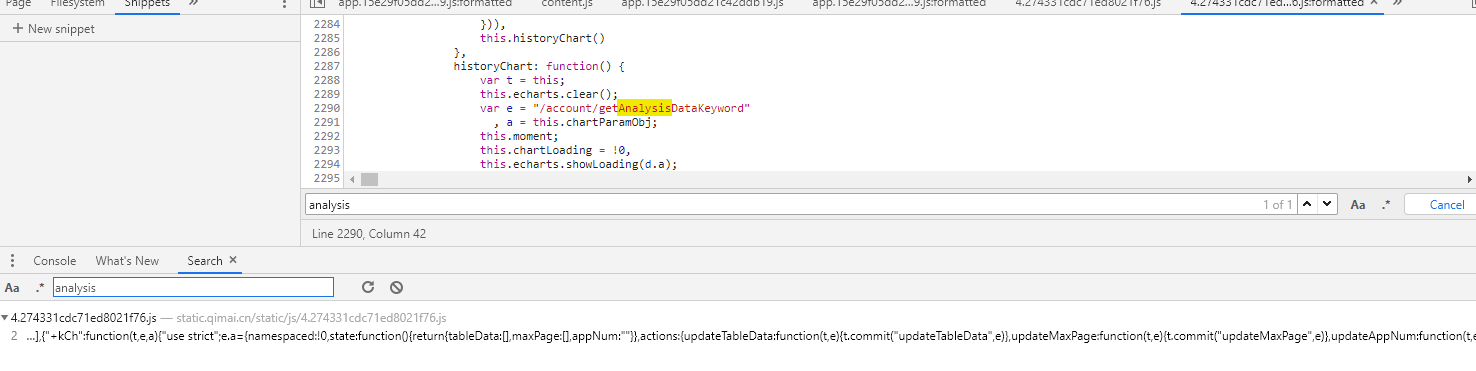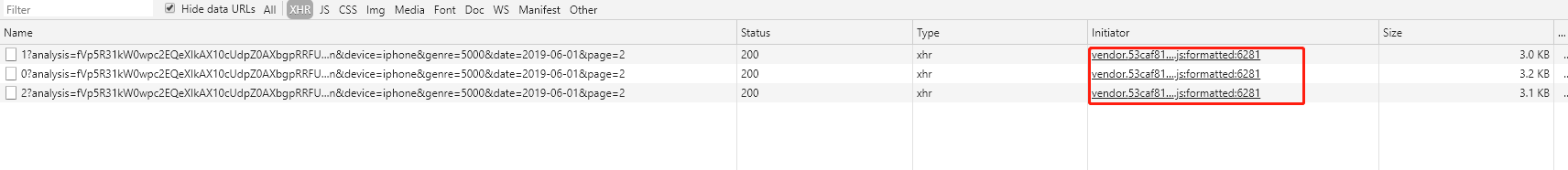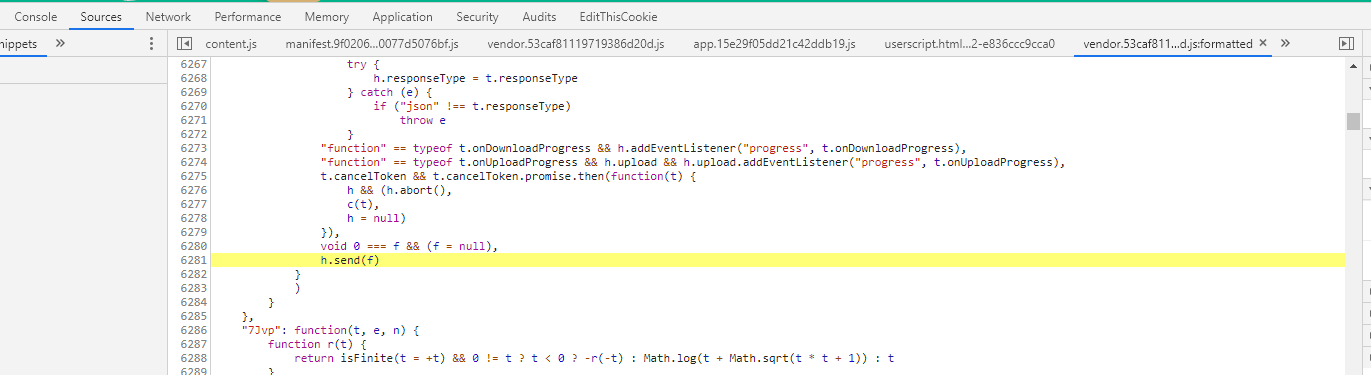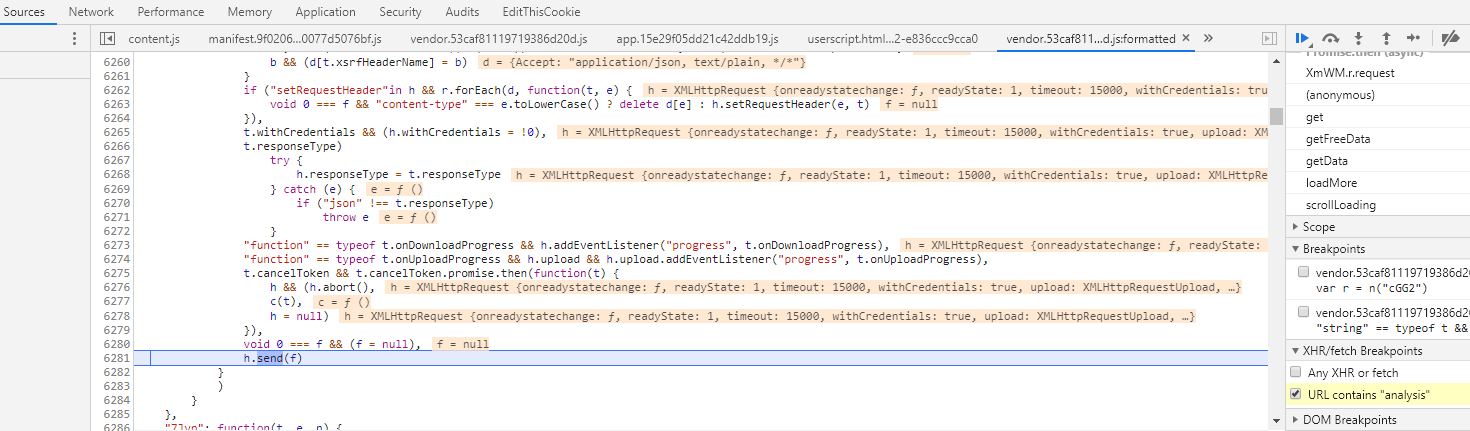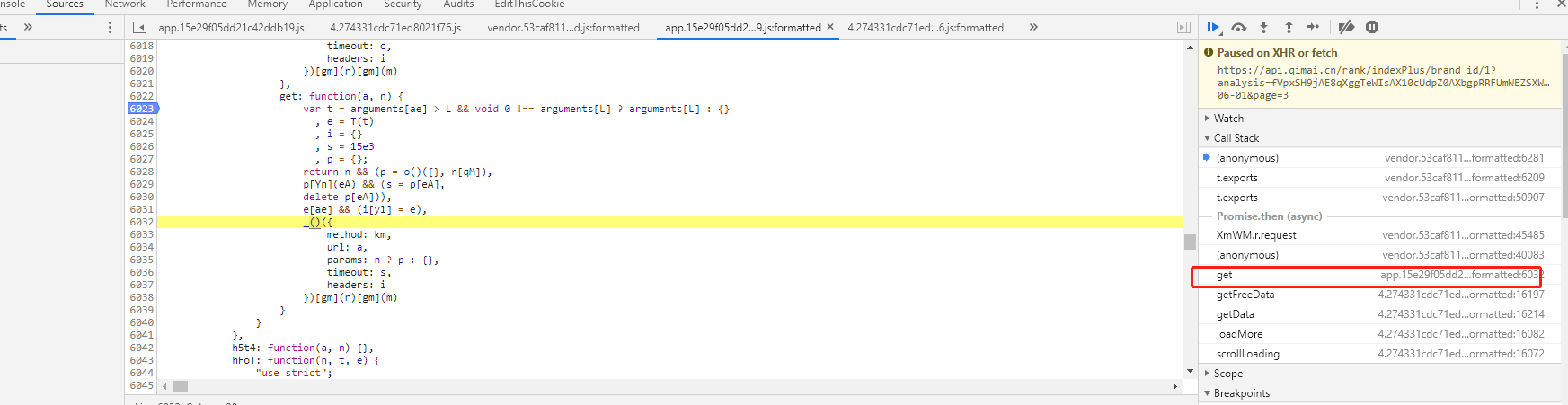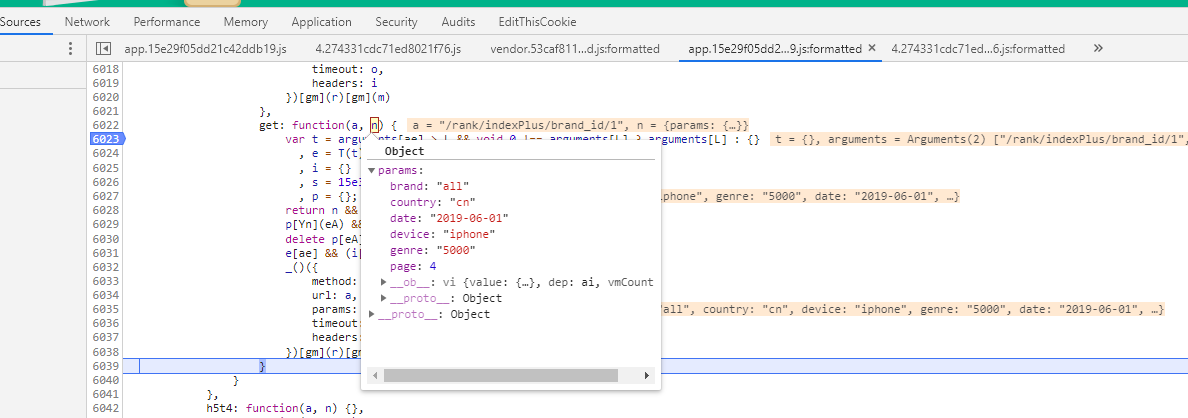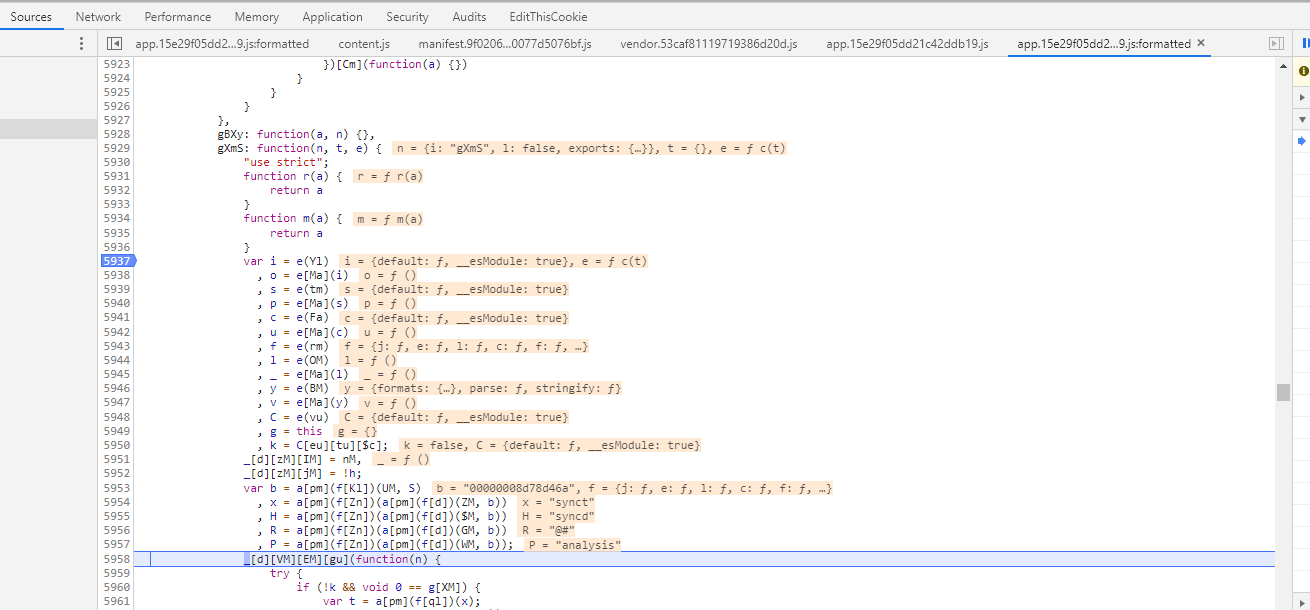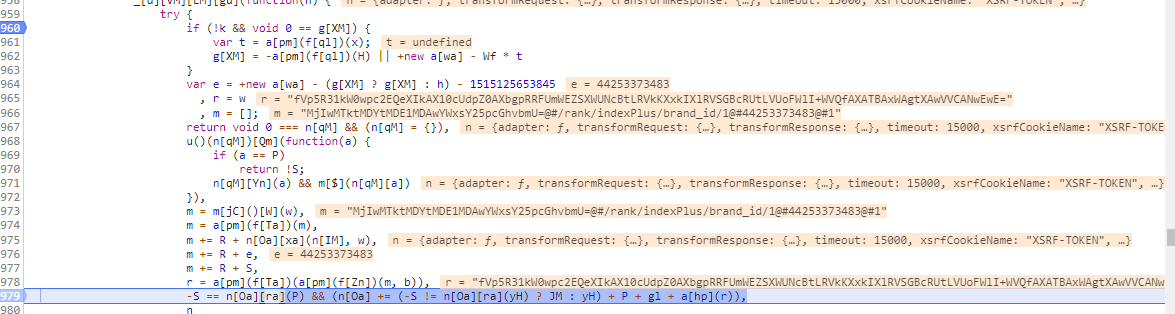### 3.加密分析

try {
if (!k && void 0 == g[XM]) {
var t = a[pm](f[ql])(x);
g[XM] = -a[pm](f[ql])(H) || +new a[wa] - Wf * t
}
var e = +new a[wa] - (g[XM] ? g[XM] : h) - 1515125653845
, r = w
, m = [];
return void 0 === n[qM] && (n[qM] = {}),
u()(n[qM])[Qm](function(a) {
if (a == P)
return !S;
n[qM][Yn](a) && m[\$](n[qM][a])
}),
m = m[jC]()[W](w),
m = a[pm](f[Ta])(m),
m += R + n[Oa][xa](n[IM], w),
m += R + e,
m += R + S,
r = a[pm](f[Ta])(a[pm](f[Zn])(m, b)),
-S == n[Oa][ra](P) && (n[Oa] += (-S != n[Oa][ra](yH) ? JM : yH) + P + gl + a[hp](r)),
n
} catch (n) {}


数据编码部分 ，通过网上查询 js 的相关知识，得知是对数据进行 Base64 编码。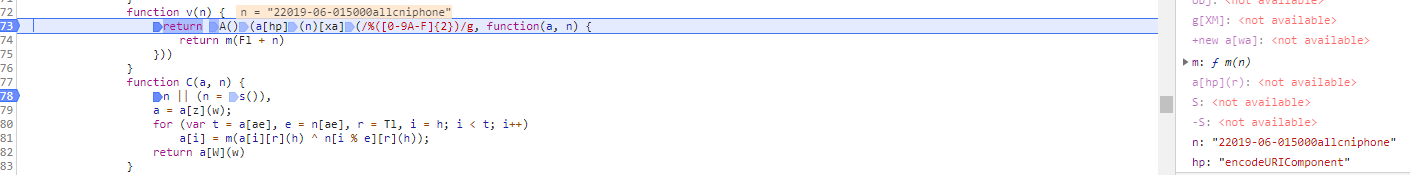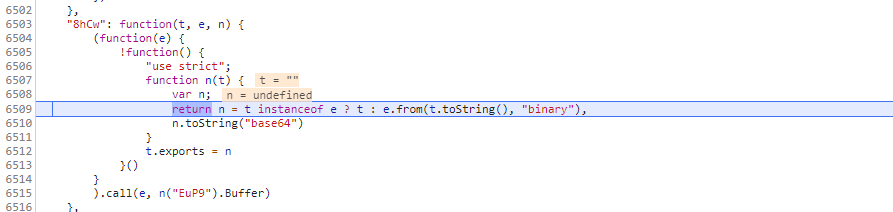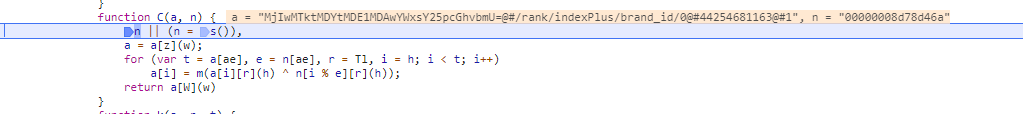• 设置一个时间差变量
• 提取查询参数值（除了 analysis）
• 排序拼接参数值字符串并 Base64 编码
• 拼接自定义字符串
• 自定义加密后再 Base64 编码
• 拼接 analysis 到 URL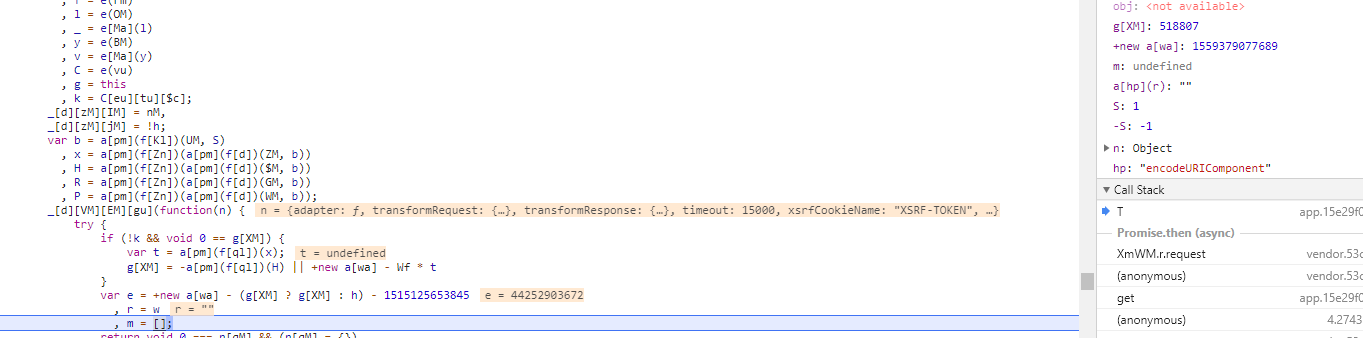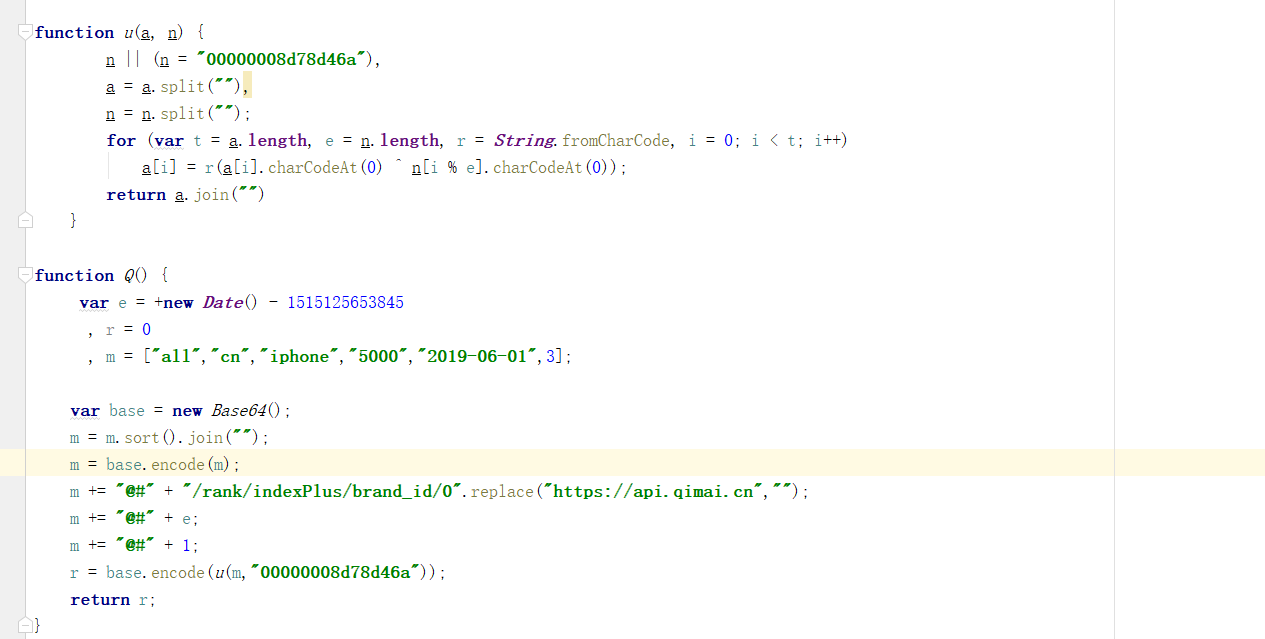base64 编码函数，是在网上查找的另外一种实现方法。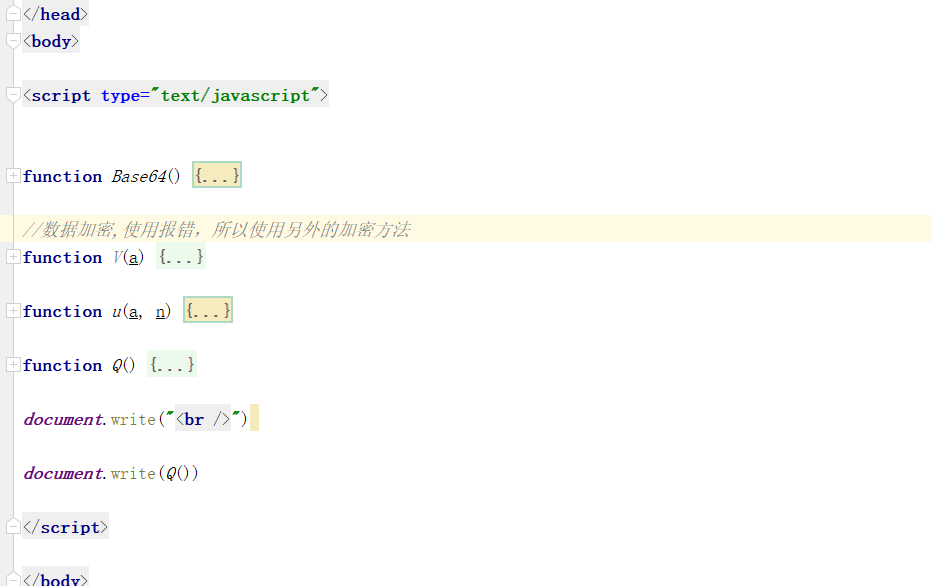## 三、Python 数据爬取

    def encrypt(self, a, n="00000008d78d46a"):
'''
加密函数，
:param a:拼接后的数据
:param n:加密时需要的数据，具有时间性，会有变化
:return:加密后的字段
'''
s = list(a)
n = list(n)
nl = len(n)
for i in range(len(s)):
#ord()函数主要用来返回对应字符的ascii码
#chr()主要用来表示ascii码对应的字符他的输入时数字
s[i] = chr(ord(s[i]) ^ ord(n[i % nl]))
return "".join(s)

def analysis(self, url):
'''
实现analysis参数数据
:param url: 三个榜单的简要url信息，形如'/rank/indexPlus/brand_id/0'，'/rank/indexPlus/brand_id/1'，'/rank/indexPlus/brand_id/2'
:return:
'''
# 提取查询参数值并排序
s = "".join(sorted([str(v) for v in self.params.values()]))
# Base64 Encode
s = base64.b64encode(bytes(s, encoding="ascii"))
# 时间差
t = str(int((time.time() * 1000) - 1515125653845))

# 拼接自定义字符串
s = "@#".join([s.decode(), url, t, "1"])

# 自定义加密 & Base64 Encode
s = base64.b64encode(bytes(self.encrypt(a=s), encoding="ascii"))
return quote(s.decode())

def run(self):
'''
爬取指定页码的APP排行信息，包括免费榜，付费榜，畅销榜
:return:
'''
for page in range(1, PAGE_NUM + 1):
self.params['page'] = page
for i in range(3):
url = self.url + str(i)
analysis = self.analysis(url)
# 拼接 URL
url_splicing = "".join([self.base_url, url, '?analysis=', analysis, '&', urlencode(self.params)])
print(url_splicing)

text = self.get_html(url_splicing)